### IMO Shortlist 2012 problem C6

Kvaliteta:
Avg: 0.0
Težina:
Avg: 8.0
The liar's guessing game is a game played between two players$A$ and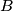$B$. The rules of the game depend on two positive integers$k$ and$n$ which are known to both players.

At the start of the game$A$ chooses integers$x$ and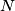$N$ with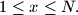$1 \le x \le N.$ Player$A$ keeps$x$ secret, and truthfully tells$N$ to player$B$. Player$B$ now tries to obtain information about$x$ by asking player$A$ questions as follows: each question consists of$B$ specifying an arbitrary set$S$ of positive integers (possibly one specified in some previous question), and asking$A$ whether$x$ belongs to$S$. Player$B$ may ask as many questions as he wishes. After each question, player$A$ must immediately answer it with yes or no, but is allowed to lie as many times as she wants; the only restriction is that, among any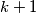$k+1$ consecutive answers, at least one answer must be truthful.

After$B$ has asked as many questions as he wants, he must specify a set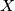$X$ of at most$n$ positive integers. If$x$ belongs to$X$, then$B$ wins; otherwise, he loses. Prove that:

1. If$n \ge 2^k,$ then$B$ can guarantee a win.
2. For all sufficiently large$k$, there exists an integer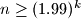$n \ge (1.99)^k$ such that$B$ cannot guarantee a win.